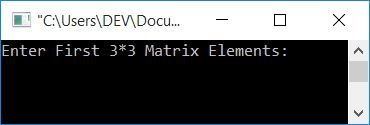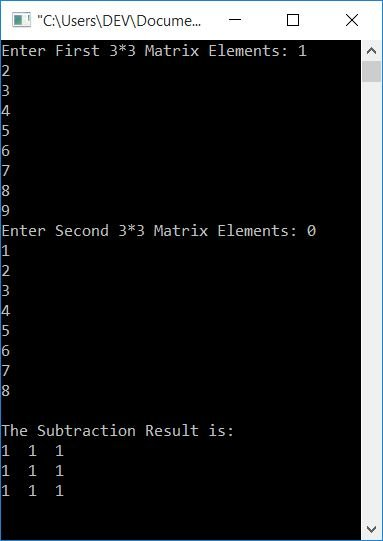# C Program to Subtract Two Matrices

In this article, you will learn and get code for matrix subtraction in C. There are two programs available here:

### How does matrix subtraction get performed?

There is a separate tutorial on it, namely, matrix subtraction. You will learn everything there is to know about matrix subtraction in a very short period of time. However, for the time being, the subtraction of any two 3*3 matrices is performed as follows:

`matSub[i][j] = mat1[i][j] - mat2[i][j];`

where i and j indicate a row and a column, respectively. When the values of i and j are set to 0:

`matSub = mat1 - mat2;`

As the above statement shows, the first number (or element) of the second matrix gets subtracted from the first number of the first matrix and initialized as the first element of the third matrix (that holds the subtraction result of both the matrices).

The number in the first matrix's 0th row and 0th column is subtracted from the number in the second matrix's 0th row and 0th column. And its subtraction result gets initialized as the value of the 0th row and 0th column of the resultant matrix. All of the elements are subtracted using the same method.

## Subtract two 3x3 matrices using a C program

To subtract two matrices in C programming, you have to ask the user to enter the two 3*3 matrices. That is, enter nine elements for the first matrix, and then nine elements for the second matrix. Now subtract the second matrix from the first. Print the subtraction result on the output as shown in the program given below.

```#include<stdio.h>
#include<conio.h>
int main()
{
int mat1, mat2, matSub, i, j;
printf("Enter First 3*3 Matrix Elements: ");
for(i=0; i<3; i++)
{
for(j=0; j<3; j++)
scanf("%d", &mat1[i][j]);
}
printf("Enter Second 3*3 Matrix Elements: ");
for(i=0; i<3; i++)
{
for(j=0; j<3; j++)
scanf("%d", &mat2[i][j]);
}
for(i=0; i<3; i++)
{
for(j=0; j<3; j++)
matSub[i][j] = mat1[i][j] - mat2[i][j];
}
printf("\nThe Subtraction Result is:\n");
for(i=0; i<3; i++)
{
for(j=0; j<3; j++)
printf("%d  ", matSub[i][j]);
printf("\n");
}
getch();
return 0;
}```

This program was built and runs in the Code::Blocks IDE. Here is its sample run:Now enter any 9 numbers for the first matrix, and then 9 numbers for the second matrix. Then press the ENTER key to see the subtraction of two given matrices. The subtraction gets performed in such a way that the first matrix gets subtracted from the second one:If you enter nine elements for the first matrix, say mat1[][]. Then these elements are stored in a way that:

• The first element gets stored at mat1.
• The second element gets stored at mat.
• The third element gets stored at mat1.
• The fourth element gets stored at mat1.
• The fifth element gets stored at mat1.
• and so on.

The same happened with the second matrix. Now, using the for loop, subtract two matrices as follows:

• The outer for loop evaluates or executes three times.
• And at every execution of the outer for loop, the inner for loop executes three times.
• As a result, the inner for loop executes three times during the first execution of the outer for loop. Therefore:
1. matSub = mat1 - mat2
2. matSub = mat1 - mat2
3. matSub = mat1 - mat2
• gets executed. The inner for loop is executed three times during the second execution of the outer for loop. Therefore again:
1. matSub = mat1 - mat2
2. matSub = mat1 - mat2
3. matSub = mat1 - mat2
• gets executed. The same things happened with the third execution:
1. matSub = mat1 - mat2
2. matSub = mat1 - mat2
3. matSub = mat1 - mat2
• As a result, the matrix matSub[][] contains the subtraction result of both the given matrix.
• Print the value of matSub[][] now.

Here  means the very first element corresponding to the matrix written before it. And  means the second element,  means the fourth element, and  means the last element.

The for loop works like this: first, and only once its initialization part executes, Then it checks the condition; if the condition evaluates to true, then the program flow executes all the statements present in the block of this for loop. Then update the loop variable, or the program flow goes to the update part. After updating the value, check the condition. If it again evaluates to be true, then program flow again does the same job of executing its block of code. The procedure is repeated until the condition evaluates to false.

## Subtract two matrices of a given size using a C program

This program has an extra feature. That is, it allows the user to define the size for both matrices.

```#include<stdio.h>
#include<conio.h>
int main()
{
int rowSize1, colSize1, rowSize2, colSize2, i, j;
int mat1, mat2, matSub;
printf("Enter Row and Column Size of First Matrix: ");
scanf("%d%d", &rowSize1, &colSize1);
printf("Enter Row and Column Size of Second Matrix: ");
scanf("%d%d", &rowSize2, &colSize2);
if(rowSize1==rowSize2 && colSize1==colSize2)
{
printf("\nEnter First %d*%d Matrix Elements: ", rowSize1, colSize1);
for(i=0; i<rowSize1; i++)
{
for(j=0; j<colSize1; j++)
scanf("%d", &mat1[i][j]);
}
printf("Enter Second %d*%d Matrix Elements: ", rowSize2, colSize2);
for(i=0; i<rowSize2; i++)
{
for(j=0; j<colSize2; j++)
scanf("%d", &mat2[i][j]);
}
printf("\nThe Subtraction Result is:\n");
for(i=0; i<rowSize1; i++)
{
for(j=0; j<colSize1; j++)
{
matSub[i][j] = mat1[i][j] - mat2[i][j];
printf("%d  ", matSub[i][j]);
}
printf("\n");
}
}
else
printf("\nSubtraction can't be Performed!");
getch();
return 0;
}```

Here is its sample run:Note: If sizes are mismatched, then subtraction can't be performed. Subtraction cannot be performed if the row size of the first matrix is not equal to the row size of the second matrix or the column size of the first matrix is not equal to the column size of the second matrix.

In the above program, at the time of subtraction, we have printed the element after each subtraction.

#### The same program in different languages

C Quiz

« Previous Program Next Program »